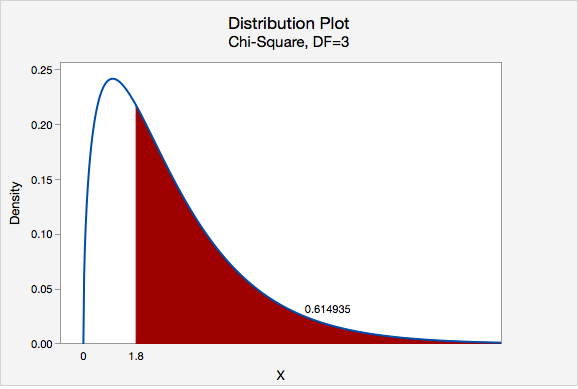# 11.2.1.2- Cards (Equal Proportions)

11.2.1.2- Cards (Equal Proportions)

## Example: Cards

Research question: When randomly selecting a card from a deck with replacement, are we equally likely to select a heart, diamond, spade, and club?

I randomly selected a card from a standard deck 40 times with replacement. I pulled 13 hearts, 8 diamonds, 8 spades, and 11 clubs.

Let's use the five-step hypothesis testing procedure:

Step 1: Check assumptions and write hypotheses

$$H_0: p_h=p_d=p_s=p_c=0.25$$
$$H_a:$$ at least one $$p_i$$ is not as specified in the null

We can use the null hypothesis to check the assumption that all expected counts are at least 5.

$$Expected\;count=n (p_i)$$

All $$p_i$$ are 0.25. $$40(0.25)=10$$, thus this assumption is met and we can approximate the sampling distribution using the chi-square distribution.

Step 2: Compute the test statistic

$$\chi^2=\sum \dfrac{(Observed-Expected)^2}{Expected}$$

All expected values are 10. Our observed values were 13, 8, 8, and 11.

$$\chi^2=\dfrac{(13-10)^2}{10}+\dfrac{(8-10)^2}{10}+\dfrac{(8-10)^2}{10}+\dfrac{(11-10)^2}{10}$$
$$\chi^2=\dfrac{9}{10}+\dfrac{4}{10}+\dfrac{4}{10}+\dfrac{1}{10}$$
$$\chi^2=1.8$$

Step 3: Determine the p-value

Our sampling distribution will be a chi-square distribution.

$$df=k-1=4-1=3$$

We can find the p-value by constructing a chi-square distribution with 3 degrees of freedom to find the area to the right of $$\chi^2=1.8$$The p-value is 0.614935

Step 4: Make a decision

$$p>0.05$$ therefore we fail to reject the null hypothesis.

Step 5: State a "real world" conclusion

There is not enough evidence to state that the proportion of hearts, diamonds, spades, and clubs that are randomly drawn from this deck are different.

  Link ↥ Has Tooltip/Popover Toggleable Visibility